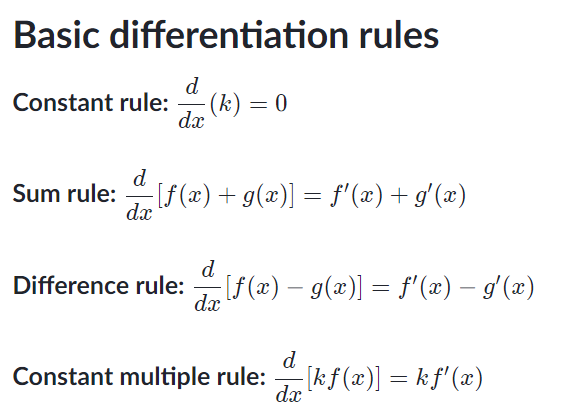# Calculus 1

Calculus 1

Formula Sheet

You will be learning about limits, derivatives, and integrals. This course is strongly based on proofs (induction, contradiction, etc.).

This also expands upon trigonometry in some ways and introduces new trigonometric terms such as sinh.

### Quick Notes:

These tend to differ based on course, textbook, or teacher… it is useful to know how other people refer to the same theorems especially if you use external resources to help you learn:

• Mean Value Theorem is also known as Lagrange’s Theorem

• Sandwich Theorem is sometimes referred to as the Squeeze Theorem

• Weierstrass’s Theorem is also known as the Extreme Value Theorem

Types of Discontinuities (makes a function not continuous):

• Type 1 Discontinuity = Jump Discontinuity (Usually setup via a piecewise defined function)

• Type 2 Discontinuity = Improper/Infinite Discontinuity (Graph usually goes to +-infinity)

``````  Example: f(x) = 1/x, then look at both one sided limits as x->0
``````
• Removable Discontinuity (A little hole in the graph at that point, the function is not defined there)

#### Graph sketching:

##### 1st derivative test – given a critical point (derivative is 0 or undefined)
• If f’(x) changes from – to + at c then F is relative minima at (c,f(c))

• If f’(x) changes from + to – is relative maxima at (c,f(c))

• If f’(x) is + or – on both sides of c, then it doesn’t mean anything

• F’(x) > 0 for all x in (a,b), then F is increasing on [a,b]

• F’(x) < 0 for all x in (a,b), then F is decreasing on [a,b]

• F’(x) = 0 for all x in (a,b), then F is constant on [a,b]

2nd derivitive test is for concave up/down

#### Derivitive Rules:• Chain Rule:

h’(x)=f’(g(x))g’(x) or h’=(f * g)’=(f’ * g) * g’

• Product Rule:

(f(x)g(x))’=f’(x)g(x)+f(x)g’(x)

• Power Rule:

f(x) = xr

f’(x) = r(x)r-1

• Quotient Rule:

(g(x)f’(x) - f(x)g’(x))/g(x)2

and g(x) != 0

3rd Party Resources:

Updated on July 6, 2023